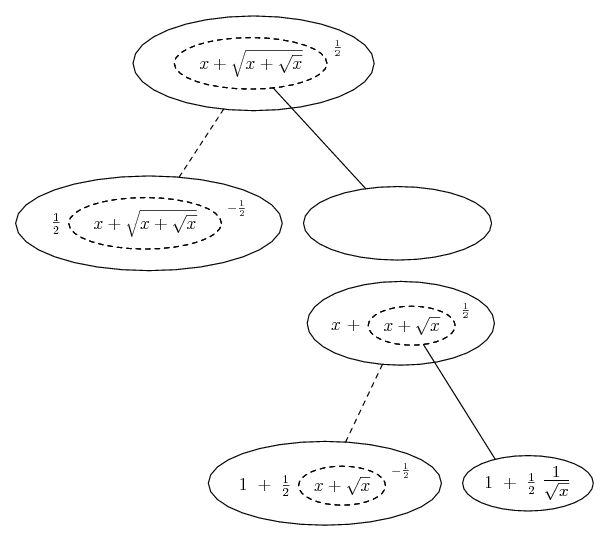# Thread: find df/dx

1. ## find df/dx

iffind

2.Originally Posted by daporeiffind
You need to use the chain rule multiple times, and set the work out carefully.

Chain rule 1: Let $\displaystyle u = x + \sqrt{x + \sqrt{x}}$.

Then $\displaystyle y = \sqrt{u}$ and $\displaystyle \frac{dy}{dx} = \frac{dy}{du} \cdot \frac{du}{dx}$.

Chain rule 2: To get $\displaystyle \frac{du}{dx}$, you will need to let $\displaystyle v = x + \sqrt{x}$.

Then $\displaystyle u = x + \sqrt{v}$ and $\displaystyle \frac{du}{dx} = 1 + \frac{d \sqrt{v}}{dx} = 1 + \frac{d \sqrt{v}}{dv} \cdot \frac{dv}{dx}$.

Now put it all together.

3. Just in case a picture helps...... because...... where... is the chain rule. Straight continuous lines differentiate downwards (integrate up) with respect to x, and the straight dashed line similarly but with respect to the dashed balloon expression (the inner function of the composite which is subject to the chain rule).

__________________________________________

Don't integrate - balloontegrate!

Balloon Calculus; standard integrals, derivatives and methods

Balloon Calculus Drawing with LaTeX and Asymptote!

4. Thank you my friends

5. ## A second method

Square both sides of the equation and then take the derivative of the resultant equation (which is actually easier to do).

df or dx